Since 12 August, 2008.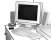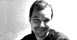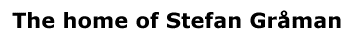# Why Strings?

While the Standard Model has been very successful in describing most of the phenomemon that we can experimentally investigate with the current generation of particle acceleraters, it leaves many unanswered questions about the fundamental nature of the universe. The goal of modern theoretical physics has been to find a "unified" description of the universe. This has historically been a very fruitful approach. For example Einstein-Maxwell theory unifies the forces of electricity and magnetism into the electromagnetic force. The Nobel prize winning work of Glashow, Salam, and Weinberg successfully showed that the electromagnetic and weak forces can be unified into a single electroweak force. There is actually some pretty strong evidence that the forces of the Standard Model should all unify as well. When we examine how the relative strengths of the strong force and electroweak force behave as we go to higher and higher energies, we find that they become the same at an energy of about 1016 GeV. In addition the gravitational force should become equally important at an energy of about 1019 GeV.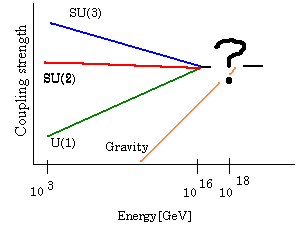The goal of string theory is to explain the "?" in the above diagram.

The characteristic energy scale for quantum gravity is called the Planck Mass, and is given in terms of Planck constant, the speed of light, and Newton's constant,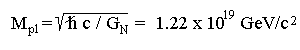Physics at this high energy scale describes the universe as it existed during the first moments of the Big Bang. These high energy scales are completely beyond the range which can be created in the particle accelerators we currently have (or will have in the foreseeable future.) Most of the physical theories that we use to understand the universe that we live in also break down at the Planck scale. However, string theory shows unique promise in being able to describe the physics of the Planck scale and the Big Bang.

In its final form string theory should be able to provide answers to answer questions like:

• Where do the four forces that we see come from?
• Why do we see the various types of particles that we do?
• Why do particles have the masses and charges that we see?
• Why do we live in 4 spacetime dimensions?
• What is the nature of spacetime and gravity?
PreviousNextContents

| Contact and Feedback |